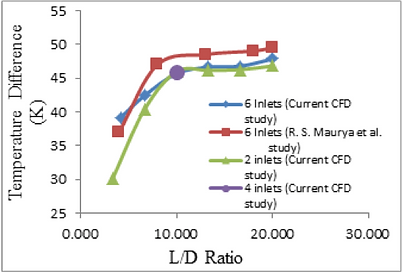## RECOVERY

Computational Evaluation of the Impact of L/D on Vortex Tube Performance

There are a number of industrial applications that require spot cooling such as cooling plastic injection moldings, setting adhesives and solders, or cooling cutting blades. One device that has been used in such applications is that of the Vortex Tube. This device relies on a compressed gas that is injected tangentially into a vortex chamber and produces a local static temperature reduction. This flow then follows a helical path as it convects downstream along the pipe wall where it experiences an increase in temperature. A portion of the hot flow is then able to escape at the end of the pipe where a conical control valve is situated. The back pressure created by the control valve returns the rest of the flow along the inner part of the tube (Figure 1).There are a number of critical geometric features of this ‘simple’ device such as

• Length to tube Diameter ratio (L/D),

• Cold Diameter to tube Diameter ratio (Dc/D),

• The number of inlet nozzles,

• Chamber Diameter to Diameter Ratio (Dch/D)

Figure 1: Diagrammatic of a Vortex Tube Flow Field

ESI has been involved in examining these parameters in order to further understand the energy separation process and in so doing, improve its performance. This has been accomplished by using a 3D computational model using the full k-ε Navier-Stokes equations.

A commercial vortex tube was acquired and computationally modeled using its actual geometry.

Figure 2 provides the time averaged total temperature contours computed within a vortex tube under given inlet pressure and temperature conditions stated by the manufacturer. These temperature contours were found to compare well with exiting published profiles on similar vortex tubes and provide a general sense of the energy separation mechanism.Figure 2: Computed Total Temperature

Validation of the computational model was achieved by experimentally testing the thermal performance of the purchased commercial vortex tube which is used for the computational model. Specifically this was achieved by comparing the computed results with an experimental evaluation of a baseline vortex tube, Figure 3, for different inlet air pressures.Figure 3:  Experimental Test SetupFigure 5:  Temperature Difference as a Function of L/D Ratio

What was a little surprising was the fact that there was little change in the flow temperatures when the cone valve inner diameter ratio (Dco/D) was altered. Figure 6 shows that only for a Dco/D = 0.5 ratio was there a small change in the hot and the cold flow exit temperatures.

The results of such a comparison is given in Figure 4 where it may be observed that there is good agreement between the experimental and computational values for the temperature difference between the hot and cold flows, especially when the inlet air supply pressure is greater than 2 Bar.Figure 4:  A Comparison of the Temperature Obtained with the CFD Model and the Experimental Results

It has also been established that as the length to diameter (L/D) ratio of the vortex tube increased, the temperature difference between the hot and cold flows also increases but quickly reaches an asymptote after L/D = 10, Figure 5.Figure 6:  Output Temperature as a Function of Dco/D Ratio Changes